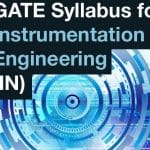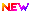# GATE 2021 Instrumentation Engineering Syllabus (Released) – Get (IN) PDF Here

Latest Applications Open 2020:GATE 2021 Syllabus of Instrumentation Engineering has been Released. GATE 2021 is a national level exam organized by IIT Bombay (IITB). The Engineering Graduation Skill Test (GATE 2021) is organized for admission to PG courses in the field of engineering and technology, specifically ME / M.Tech.

It’s a Graduate aptitude test for engineering. The Scores from GATE can help to get admissions in M. Tech/Ph.D. programs in multiple sectors like IIT’s & IISER’s.

GATE exams are held every year on a rotational basis by the IIT’s & IISC in multiple zones. Not only for the examination but GATE Scores is also plays an essential role in the recruitment of the Government and private organizations who are in the technical field.

## GATE 2021 Instrumentation Engineering Syllabus – PDF ReleasedGATE 2021 Instrumentation Engineering Syllabus has been Released. Click Here to Download Pdf.

## Section 1: Engineering Mathematics

Latest Applications For Various UG & PG Courses Open 2020:

1. Manav Rachna University, Haryana – 2020 UG & PG Admission Open. Apply Now
2. Bennett University (Times Group), Admission Open for 2020. Apply Now
3. Chandigarh University, Punjab 2020 Admission Open for all Courses. Apply Now
4. MIT World Peace University, Admissions Open for All Courses 2019-20. Apply Now
5. St. Andrew’s Institute of Technology and Management, Admission open 2020. Apply Now

Linear Algebra: Matrix algebra, systems of linear equations, Eigenvalues and Eigenvectors.

Calculus: Mean value theorems, theorems of integral calculus, partial derivatives, maxima and minima, multiple integrals, Fourier series, vector identities, line, surface and volume integrals, Stokes, Gauss and Green’s theorems.

Differential equations: First order equation (linear and nonlinear), higher-order linear differential equations with constant coefficients, Method of variation of parameters, Cauchy’s and Euler’s equations, Initial and boundary value problems, the solution of partial differential equations: variable separable method.

Analysis of complex variables: Analytic functions, Cauchy’s integral theorem, and integral formula, Taylor’s and Laurent’s series, residue theorem, a solution of integrals.

Probability and Statistics: Sampling theorems, conditional probability, mean, median, mode and standard deviation, random variables, discrete and continuous distributions: normal, Poisson and binomial distributions.

Numerical Methods: Matrix inversion, solutions of non-linear algebraic equations, iterative methods for solving differential equations, numerical integration, regression and correlation analysis.

## Section 2: Electrical Circuits:

Voltage and current sources: independent, dependent, ideal and practical; v-i relationships of resistor, inductor, mutual inductor, and the capacitor; transient analysis of RLC circuits with dc excitation.

Kirchoff’s laws, mesh and nodal analysis, superposition, Thevenin, Norton, maximum power transfer, and reciprocity theorems.

Peak-, average- and RMS values of ac quantities: apparent-, active- and reactive powers; phasor analysis, impedance, and admittance; series and parallel resonance, locus diagrams, a realization of basic filters with R, L and C elements.

One-port and two-port networks, driving point impedance and admittance, open-, and short circuit parameters.

## Section 3: Signals and Systems

Periodic, aperiodic and impulse signals; Laplace, Fourier, and z-transforms; transfer function, the frequency response of first and second-order linear time-invariant systems, an impulse response of systems; convolution, correlation. Discrete-time system: impulse response, frequency response, pulse transfer function; DFT and FFT; basics of IIR and FIR filters.

## Section 4: Control Systems

Feedback principles, signal flow graphs, transient response, steady-state-errors, Bode plot, phase and gain margins, Routh and Nyquist criteria, root loci, design of lead, lag and lead-lag compensators, state-space representation of systems; time-delay systems; mechanical, hydraulic and pneumatic system components, synchro pair, servo, and stepper motors, servo valves; on-off, P, P-I, P-I-D, cascade, feedforward, and ratio controllers.

## Section 5: Analog Electronics

Characteristics and applications of the diode, Zener diode, BJT and MOSFET; small-signal analysis of transistor circuits, feedback amplifiers. Characteristics of operational amplifiers; applications of opamps: difference amplifier, adder, subtractor, integrator, differentiator, instrumentation amplifier, precision rectifier, active filters, and other circuits. Oscillators, signal generators, voltage-controlled oscillators, and phase-locked loop.

## Section 6: Digital Electronics

Combinational logic circuits, minimization of Boolean functions. IC families: TTL and CMOS. Arithmetic circuits, comparators, Schmitt trigger, multi-vibrators, sequential circuits, flip-flops, shift registers, timers, and counters; sample-and-hold circuit, multiplexer, analog-to-digital (successive approximation, integrating, flash and sigma-delta) and digital-to-analog converters (weighted R, R-2R ladder and current steering logic). Characteristics of ADC and DAC (resolution, quantization, significant bits, conversion/settling time); basics of number systems, an 8-bit microprocessor, and microcontroller: applications, memory, and input-output interfacing; basics of data acquisition systems.

## Section 7: Measurements

SI units, systematic and random errors in measurement, expression of uncertainty – accuracy and precision index, propagation of errors. PMMC, MI and dynamometer type instruments; dc potentiometer; bridges for measurement of R, L, and C, Q-meter. Measurement of voltage, current, and power in single and three-phase circuits; ac and dc current probes; true RMS meters, voltage and current scaling, instrument transformers, timer/counter, time, phase and frequency measurements, digital voltmeter, digital multimeter; oscilloscope, shielding, and grounding.

## Section 8: Sensors and Industrial Instrumentation

Resistive-, capacitive-, inductive-, piezoelectric-, Hall effect sensors and associated signal conditioning circuits; transducers for industrial instrumentation: displacement (linear and angular), velocity, acceleration, force, torque, vibration, shock, pressure (including low pressure), flow (differential pressure, variable area, electromagnetic, ultrasonic, turbine, and open channel flow meters) temperature (thermocouple, bolometer, RTD (3/4 wire), thermistor, pyrometer, and semiconductor); liquid level, pH, conductivity and viscosity measurement.

## Section 9: Communication and Optical Instrumentation

Latest Applications For Various UG & PG Courses Open 2020:

1. Manav Rachna University, Haryana – 2020 UG & PG Admission Open. Apply Now
2. Bennett University (Times Group), Admission Open for 2020. Apply Now
3. Chandigarh University, Punjab 2020 Admission Open for all Courses. Apply Now
4. MIT World Peace University, Admissions Open for All Courses 2019-20. Apply Now
5. St. Andrew’s Institute of Technology and Management, Admission open 2020. Apply Now

Amplitude- and frequency modulation and demodulation; Shannon’s sampling theorem, pulse code modulation; frequency and time division multiplexing, amplitude-, phase-, frequency-, pulse shift keying for digital modulation; optical sources and detectors: LED, laser, photo-diode, light-dependent resistor, and their characteristics; interferometer: applications in metrology; basics of fiber optic sensing.

If you any query regarding the GATE 2021 Instrumentation Engineering Syllabus, you can ask your query leave comments below.Manav Rachna UniversityMIT WPU

+